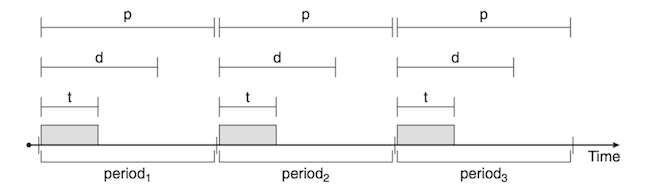## Real Time Scheduling Algorithms

#### Introduction:

Real time operating system is a system that must meet the time constrains for a computational process and also the correctness of the result, if not, system failure can exist. These constrains vary from the aspect of importance such as safety related task is more important than data-logging task. Flight systems, medical devices, and high speed multimedia communication are examples of real time systems.

Real time systems can be categorized into hard real time system in which task must be executed by its deadline under all conditions (such as medical apps), and soft real time system in which task may miss some deadlines and the system could work correctly (such as audio or video streaming).

#### Real time scheduling algorithms:

In a system of set of processes, determining which process should be executed next in addition to satisfying the time constrains are the main problem for the scheduler. Real time system must respond to the event as quickly as possible, when an event is occurred. There are latencies that can affect the performance of this system and they must be reduced.

Latency Definition
Event latency The period of time between the occurrence and the response it event latency.
Interrupt latency The period of time between the arrival of an interrupt at the CPU and the start of its ISR (interrupt service routine).
Dispatch latency The amount of time required for the scheduling dispatcher to stop one process and start another.

As the real time operation system must response immediately to the real time processes, the scheduler in such systems must support a priority-based algorithms with preemption, such that the more important tasks are assigned higher priorities than those are less important, and the CPU will be preempted if a higher priority process available to run.

Processes to be scheduled must have some characteristics.

1. It must be periodic, it requires the CPU at constant intervals period p.
2. It has processing time t.
3. It has a deadline it must be serviced before it.

The relationship of the processing time, the deadline, and the period can be expressed as 0 ≤ t ≤ d ≤ p.#### Rate Monotonic Scheduling :

It is a uniprocessor static-priority preemptive algorithm. In this schema, shorter period task is assigned as a higher priority tasks, and the longer period task assigned as a lower priority tasks, rather than assigning priorities at runtime.

Simple to understand. Lower CPU utilization.
Easy to implement. It deals only with independent tasks.
Though some of the lower priority tasks fail to meet

##### Example :

Consider two processes P1 and P2 where p1 = 50, t1=20, p2 =100, and t2=35.
Can these processes be scheduled using rate-monotonic (RMS) scheduling? Illustrate your answer using Gantt chart

##### Example :

Consider two processes P1 and P2 where p1 = 50, t1=25, p2 =75, and t2=30.
Can these processes be scheduled using rate-monotonic (RMS) scheduling? Illustrate your answer using Gantt chart

It is a dynamic priority algorithm so that the priority of a task can change during runtime. The highest priority job is the one with the earliest deadline, If two tasks have the same absolute deadlines, chose one of them randomly.

Optimal algorithm. Difficult to implement due to the dynamic priority assignment.
Best CPU untilization. It introduces a large runtime overhead, because deadlines need to be updated from a job to the other.

##### Example :

Consider two processes P1 and P2 where p1 = 50, t1=25, p2 =75, and t2=30.
Illustrate the scheduling of these two processes using earliest-deadline-first (EDF) scheduling.

Share: